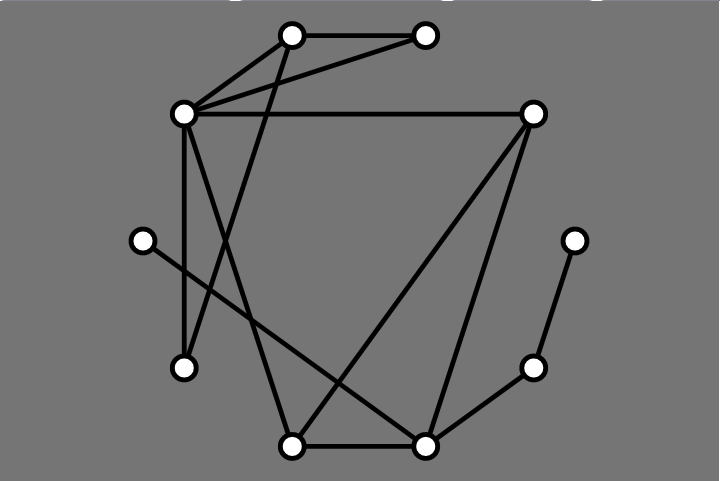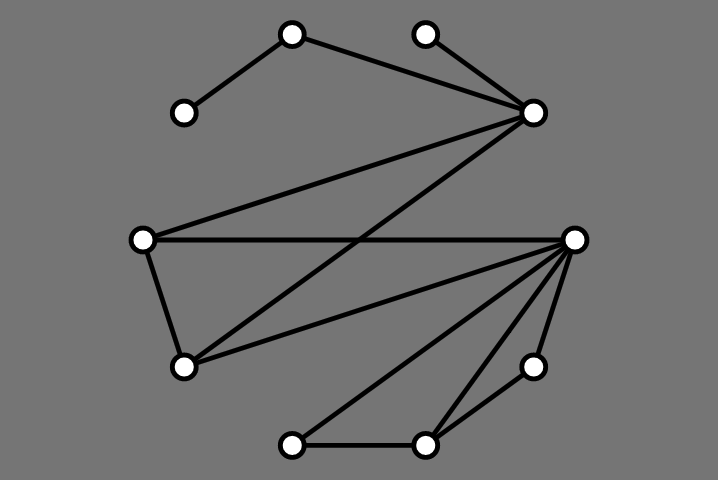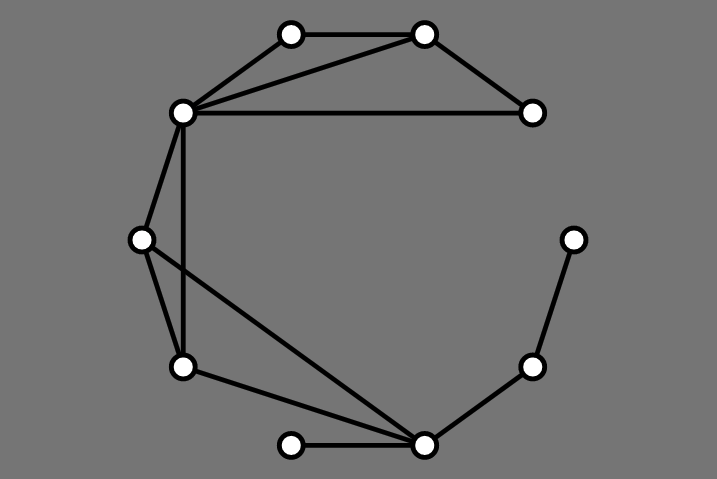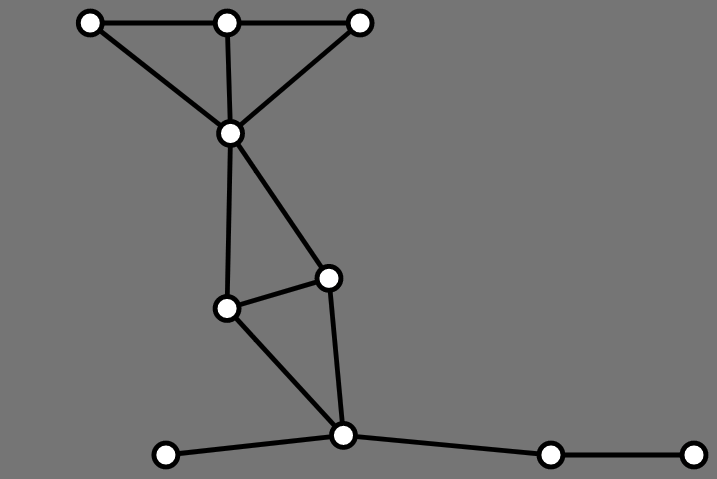# Lecture 16: Drawing General Graphs II

## Announcements

1. Assignment 07 posted, due Friday
2. Quiz Next Monday
• Apply Tidy Tree algorithm by hand
3. Assignment 08 posted soon, due next Friday
4. Limited OH This Week (advising week)
• No OH today
• No OH on Thursday

## Outline

1. AVSDF Circular Layouts
2. Force-directed Graph Layout

## Goals

From Fruchterman and Reingold (1991):

1. Distribute the vertices evenly in the frame.
2. Minimize edge crossings.
3. Make edge lengths uniform.
4. Reflect inherent symmetry.
5. Conform to the frame.

## Last Time: Circular LayoutsSimpler Challenge: pick an order for vertices

## Mäkinen Procedure

1. Find two vertices of highest degree and add them to left/right sets
2. Repeat until all vertices are added to left or right:
• compute (right neighbors) - (left neighbors) for each vertex
• add vertex with largest value to right
• add vertex with smallest value to left
3. Add left vertices on left side, right on right side

## Mäkinen Results: Before## Mäkinen Results: After## AVSDF Heuristic

• He & Sykora

Idea:

• perform depth-first search, starting from vertex of minimal degree
• always explore minimum degree neighbor first

## AVSDF Example

1: 2, 6, 3, 5
2: 1, 3, 5, 6
3: 1, 2, 6
4: 2, 5
5: 1, 2, 4
6: 1, 3


## How To Implement AVSDF Efficiently

• What do we keep track of and store?
• How do we update data structures?
• How efficient is the procedure

## AVSDF Initialization

	const order = [];
const stack = [];
const vertices = this.graph.vertices;
const n = vertices.length;
const placed = new Array(n).fill(false);

vertices.sort((u, v) => {
return u.degree() - v.degree();
});

stack.push(vertices);


## Main Loop

while (stack.length > 0) {
let vtx = stack.pop();
if (!placed[vtx.id]) {
order.push(vtx);
placed[vtx.id] = true;
vtx.neighbors.sort((u, v) => {
return v.degree() - u.degree();
});
for (let nbr of vtx.neighbors) {
if (!placed[nbr.id]) { stack.push(nbr); }
}}}


## 1: Random Circular## 2: Mäkinen Circular## 3: AVSDF Circular# Force-Directed Layout

## A Different Approach

Don’t place vertices explicitly

• associate graph with a physical system
• simulate the physical system
• let system evolve
• place vertices at final location according to evolution

## Goals, Again

From Fruchterman and Reingold (1991):

1. Distribute the vertices evenly in the frame.
2. Minimize edge crossings.
3. Make edge lengths uniform.
4. Reflect inherent symmetry.
5. Conform to the frame.

## Physical Simulation

1. All vertices should repel each other

2. Adjacent vertices should attract each other

Due to:

• Fruchterman and Reingold, 1991

## Competing Forces

All vertices:

function repulsiveForce (dist, k) {
return k * k / dist;
}

function attractiveForce (dist, k) {
return dist * dist / k;
}


## Question

When do attractive and repulsive forces cancel out for adjacent vertices?

function repulsiveForce (dist, k) {
return k * k / dist;
}

function attractiveForce (dist, k) {
return dist * dist / k;
}


## F&R Main Loop

For each vertex:

• compute net force on that vertex
• find repulsive contribution from each other vertex
• find attractive contribution from each neighbor
• sum all contributions
• move each vertex according to net force
• move in direction of net force
• amount is min of net force and “temperature”
• update temperature

Repeat until “done”

## Setting Parameters

Want k is “ideal” distance between vertices

• area = width * height
• n = vertices.length
• k = C * Math.sqrt(area / n)
• k is “typical” distance if vertices are spread out
• C some constant to be determined

## Computing Forces I

• v at point (v.x, v.y)
• u at point (u.x, u.y)

What is distance from v to u?

• deltaX = v.x - u.x
• deltaY = v.y - u.y
• delta = Math.sqrt(deltaX * deltaX + deltaY * deltaY)

## Computing Forces II

Want (repsulive) force in direction of (deltaX, deltaY) with given amount (length): repulsiveForce(delta, k)

How to get this?

• dx = (deltaX / delta) * repulsiveForce(delta, k)
• dy = (deltaY / delta) * repulsiveForce(delta, k)

for (let v of vertices) {
dx[v.id] = 0; dy[v.id] = 0;
for (let u of vertices) {
if (v != u) {
let deltaX = v.x - u.x;
let deltaY = v.y - u.y;
let delta = Math.sqrt(deltaX * deltaX + deltaY * deltaY);
dx[v.id] += (deltaX / delta) * repulsiveForce(delta, k);
dy[v.id] += (deltaY / delta) * repulsiveForce(delta, k);
}}}


## Similarly For Attractive Forces

for (let e of edges) {
let v = e.vtx1; let u = e.vtx2;
let deltaX = v.x - u.x; let deltaY = v.y - u.y;
let delta = Math.sqrt(deltaX * deltaX + deltaY * deltaY);
dx[v.id] -= (deltaX / delta) * attractiveForce(delta, k);
dy[v.id] -= (deltaY / delta) * attractiveForce(delta, k);
dx[u.id] += (deltaX / delta) * attractiveForce(delta, k);
dy[u.id] += (deltaY / delta) * attractiveForce(delta, k);
}


## Applying Forces

for (let v of vertices) {
let d = Math.sqrt(dx[v.id] * dx[v.id] + dy[v.id] * dy[v.id]);
v.x += (dx[v.id] / d) * Math.min(d, temp);
v.y += (dy[v.id] / d) * Math.min(d, temp);
v.x = Math.max(v.x, xMin);
v.x = Math.min(v.x, xMax);
v.y = Math.max(v.y, yMin);
v.y = Math.min(v.y, yMax);
}


## Finally

Repeat:

• update positions
• decrease temperature

Stop when temperature is 0 (or some fixed number of iterations)

## 1: Random Circular## 2: Mäkinen Circular## 3: AVSDF Circular## 4: Force Directed## Okay

But it is WAY BETTER with animation

• Demo: lec16-graph-drawing.zip